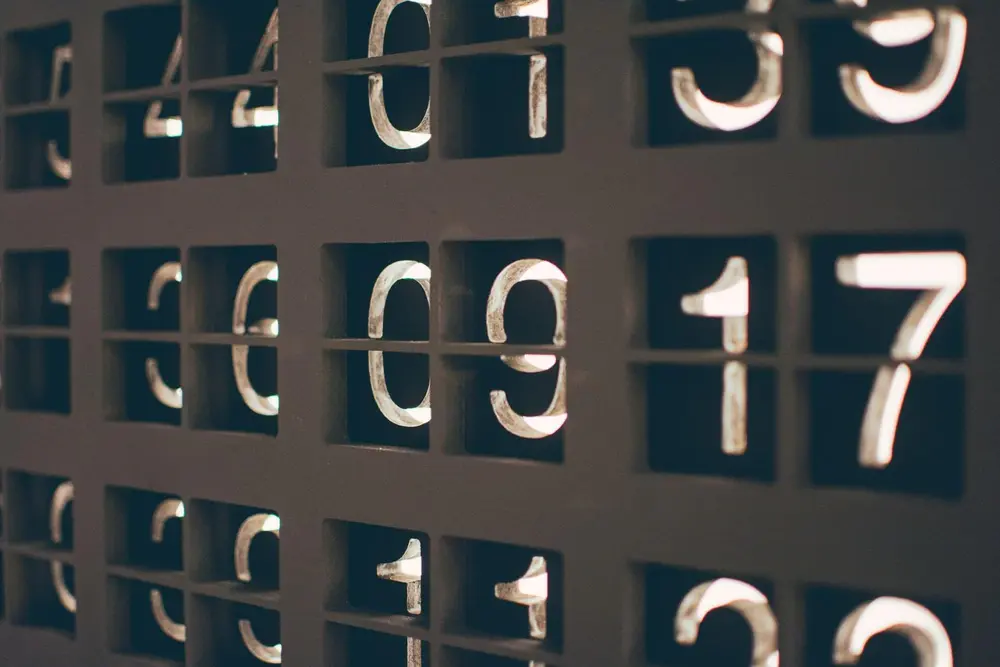# Accuracy

Numbers can contain a certain amount of error. Accuracy measures the amount of error or uses the known amount of error to find maximum or minimum possible values.

You can work out the percentage error by:

( The error ÷ The real value ) × 100

For example:
( ( 5.22 - 5.2 ) ÷ 5.22 ) × 100 = 0.38%

Numbers are not always exact. Sometimes numbers are given which are just an average or correct to a certain degree of accuracy. This means the number provided would have a minimum and a maximum possible value. This is shown below:

2.4 given to 1 decimal place means:
The minimum is 2.35 as this rounded to 1 decimal place is 2.4
The maximum is just under 2.45 as this rounded to 1 decimal place is 2.4

10m given to the nearest metre means:
The minimum is 9.5m as this rounded to the nearest metre is 10m.
The maximum is anything up to 10.5m as this would round to 10m to the nearest metre.

1.38 given to 3 significant numbers means:
The range is 1.375 up to 1.385.

Number

Number

Number

Number

Number

Number